# Projectile MotionPage 3

#### WATCH ALL SLIDES

CONCLUSIONS:

Horizontal range is greatest for the throw angle of 450

Horizontal ranges are the same for angles Θ and (900 – Θ)

Slide 28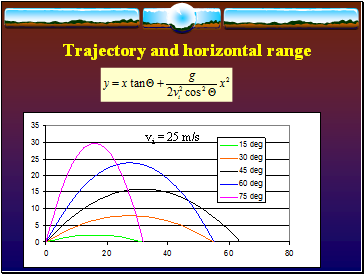Trajectory and horizontal range

vi = 25 m/s

Slide 29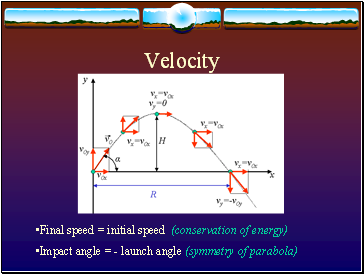Velocity

Final speed = initial speed (conservation of energy)

Impact angle = - launch angle (symmetry of parabola)

Slide 30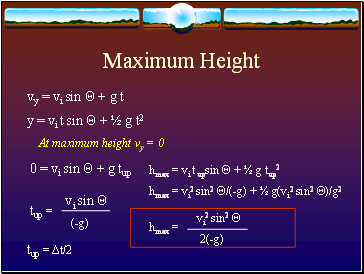## Maximum Height

vy = vi sin Θ + g t

y = vi t sin Θ + ½ g t2

At maximum height vy = 0

0 = vi sin Θ + g tup

tup = Δt/2

Slide 31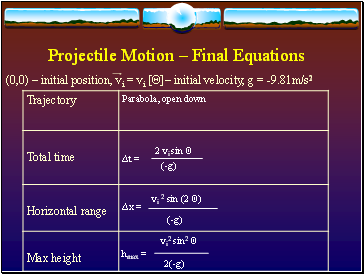## Projectile Motion – Final Equations

(0,0) – initial position, vi = vi [Θ]– initial velocity, g = -9.81m/s2

2 vi sin Θ

(-g)

vi2 sin2 Θ

2(-g)

Slide 32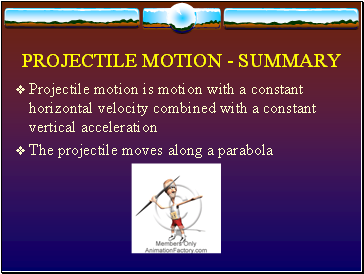## Projectile motion - summary

Projectile motion is motion with a constant horizontal velocity combined with a constant vertical acceleration

The projectile moves along a parabola

Go to page:
1  2  3